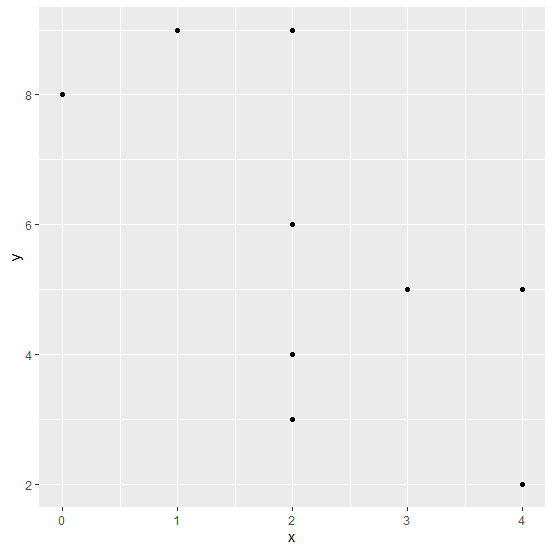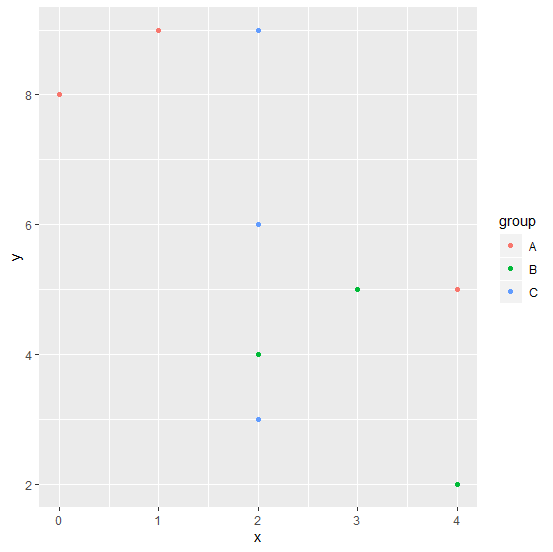# How to create a scatterplot with colors of the group of points in R?

A scatterplot is the plot that has one dependent variable plotted on Y-axis and one independent variable plotted on X-axis. Sometimes the pair of dependent and independent variable are grouped with some characteristics, thus, we might want to create the scatterplot with different colors of the group based on characteristics. For this purpose, we can use colour argument in ggplot function.

## Example

Consider the below data frame −

set.seed(123)
x <-rpois(10,2)
y <-rpois(10,5)
group <-c("A","B","C","A","A","A","C","B","B","C")
df <-data.frame(x,y,group)
df

## Output

x y group
1 1 9 A
2 3 5 B
3 2 6 C
4 4 5 A
5 4 2 A
6 0 8 A
7 2 3 C
8 4 2 B
9 2 4 B
10 2 9 C

## Example

Creating a simple scatterplot −

library(ggplot2)
ggplot(df,aes(x,y))+geom_point()

## OutputCreating a scatterplot with color of points based on groups −

ggplot(df,aes(x,y,colour=group))+geom_point()

## Output